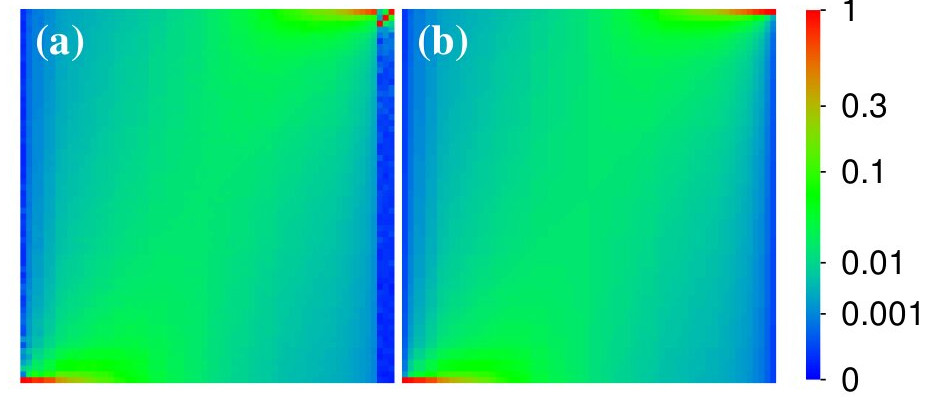Nonlinear perturbation of Random Matrix Theory
by K.M.Frahm and D.L.Shepelyansky
arXiv:2212.11955[cond-mat.stat-mech]Density plot of linear mode probability shown by color: (a) numerical data, (b) theory from energy equipartition anzats; beta=1, size N=64; x-axis: initial mode number, y-axis: detected mode number

 ` `Article download (22 Dec 2022, here) , version v2 (17 May 2023, here)Article published Phys. Rev. Lett. v.131. p.077201 (2023) (here) [no Refs. misprints in supmat]Additional figures in zip file (here) and (README.txt)Updates 15 Feb 2023 (lines below)Matrix size N=256 (N256a, N256b, N256c, N256d) and (READMEupdate.txt see update point 2)Lyapunov exponents at large times (LyapunovA, LyapunovB, LyapunovC, LyapunovD) and (READMEupdate.txt see update points respectively 6-9)All Additional figures with updates 15 Feb 2023 in zip file (here) and (READMEupdate.txt)NOTE ADDED: after 22 Dec 2022 submission of our work there appeared PRL publication "Observation of Light Thermalization to Negative-Temperature Rayleigh-Jeans Equilibrium States in Multimode Optical Fibers" by K. Baudi, J. Garnier, A. Fusaro, N. Berti, C. Michel, K. Krupa, G. Millot, A. Picozzi Phys. Rev. Lett. v.130, p.063601 (2023), dated by 8 Feb 2023: negative temperature energy equipartition distribution (EQ in Eq.(2) in our work or Rayleigh-Jeans in PRL) is experimentally observed with 45 modes in optical fiber, even if initial linear spectrum of modes is Em = (m1+m2+const) different from RMT, a dynamical thermalization is still present. This webpage is created at December 22, 2022 and is maintained by K.M.Frahm and D.L.Shepelyansky